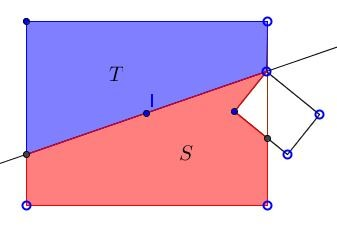# Cutting a Hole in Half

### Problem

Given a rectangle with a rectangular hole.Cut the shape into two of equal areas with one straight line cut.

### Solution

The solution is extremely simple and may be discerned from the following figure:Due to the central symmetry of a rectangle, the line joining the centers of the two rectangles cuts both of them into equal parts and therefore solves the problem.

In the previous sentence I have intentionally referred to two rectangles and not to a rectangle and a hole. This is because the same line that joins the centers of the two rectangles solves another problem, viz., cutting two now disjoint rectangles, each into equal halves with a single straight line:### A counterexample to a generalization

Given that the same procedure worked equally well when a smaller rectangle was inside or entirely outside the bigger one, it is naturally to ask whether that approach also works when the two rectangles intersect but not include one another.

The answer to that inquiry is negative, "No, the line joining the centers of the two rectangles does not always halve their difference (nor their union.)" Below there is a counterexample to this conjecture.The small rectangle intersects the bigger one only above the line joining their centers such that the two pieces of the bigger rectangle cannot have equal areas.

### A working generalization

So, given this shape - the difference of two intersecting but not overlapping rectangles, we may still inquire whether there is a straight line that cuts that shape into two of equal areas. The answer to that is that there is a multitude of ways of doing that, e.g., the line may be required to pass through an arbitrary point or be parallel to an arbitrary direction. The same underlying reasoning applies in both case, to more general shapes, and even when the task is to cut simultaneously two arbitrary shapes. (The latter case is known as the Ham sandwich theorem.)

Let $[X]$ stands for the area of shape $X.$ The fundamental assumption that underlies our argument is that $[X]$ is a continuous function of $X$ by which I mean that a "small change" in $X$ results in a small change in $[X].$ For continuous function we have Bolzano's theorem which is also known as the Intermediate Value Theorem:

If function $f$ continuous in a closed interval $[a,b]$ attains values of different signs at the endpoints of the interval $(f(a)f(b)\lt 0)$ then there is a point $c\in (a,b)$ where the function vanishes $f(c)=0.$

To see how this works, draw an arbitrary line through a given point $(I$ in the diagram below) and choose a direction on that line. The line cuts the given shape into two, one to the left of the line, say $S,$ the other $T$ to the right.Note the sign of the difference $[S]-[T].$ (If the difference is $0$ this is the line we've been looking for.) Now rotate (slowly if you will) the line through the chosen point. A rotation through a small angle will change just a little both $S$ and $T$ and, therefore, also $[S]-[T].$Remember that $S$ is that portion of the given shape that lies to the left of the line, $T$ lies to the right. Now the punch line: after a rotation through $180^{\circ}$ $S$ and $T$ become swapped and, therefore, their difference changes its sign. Thus if we consider $[S]-[T]$ as a function of angle starting with an arbitrary position: $f(\alpha )= [S]-[T]$ then $f(0^{\circ})$ and $f(180^{\circ})$ have different signs, so that Bolzano's theorem allows us to conclude that somewhere along the way the difference in the areas $[S]-[T]$ was $0$ - just what we wanted to prove.

### References

1. M. Chamberland, Single Digits. In Praise of Small Numbers, Princeton University Press, 2015.